Examples

Chapter 4 Class 11 Mathematical Induction
Serial order wise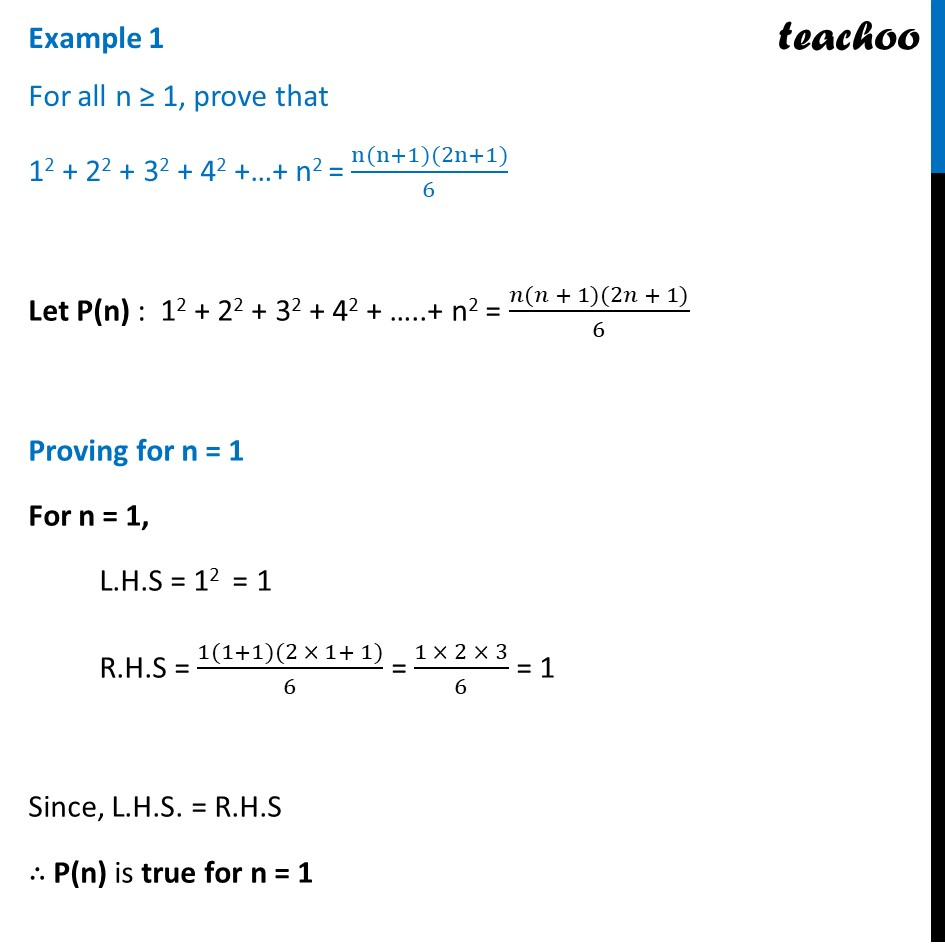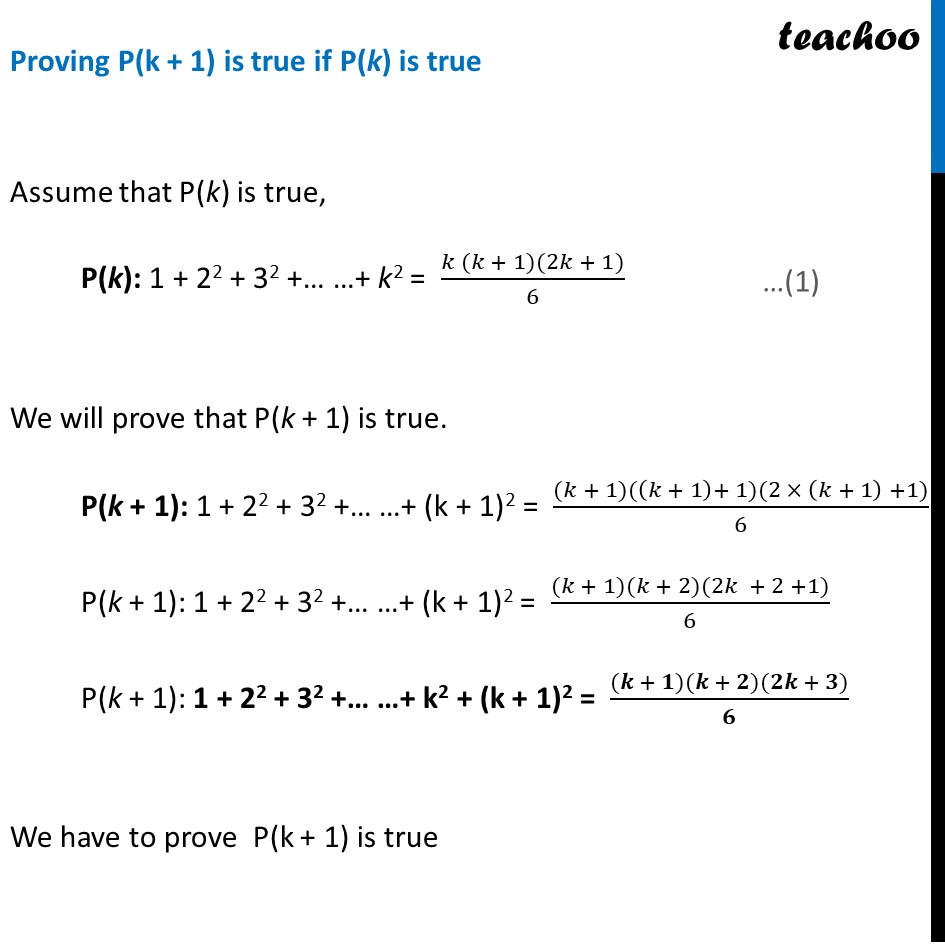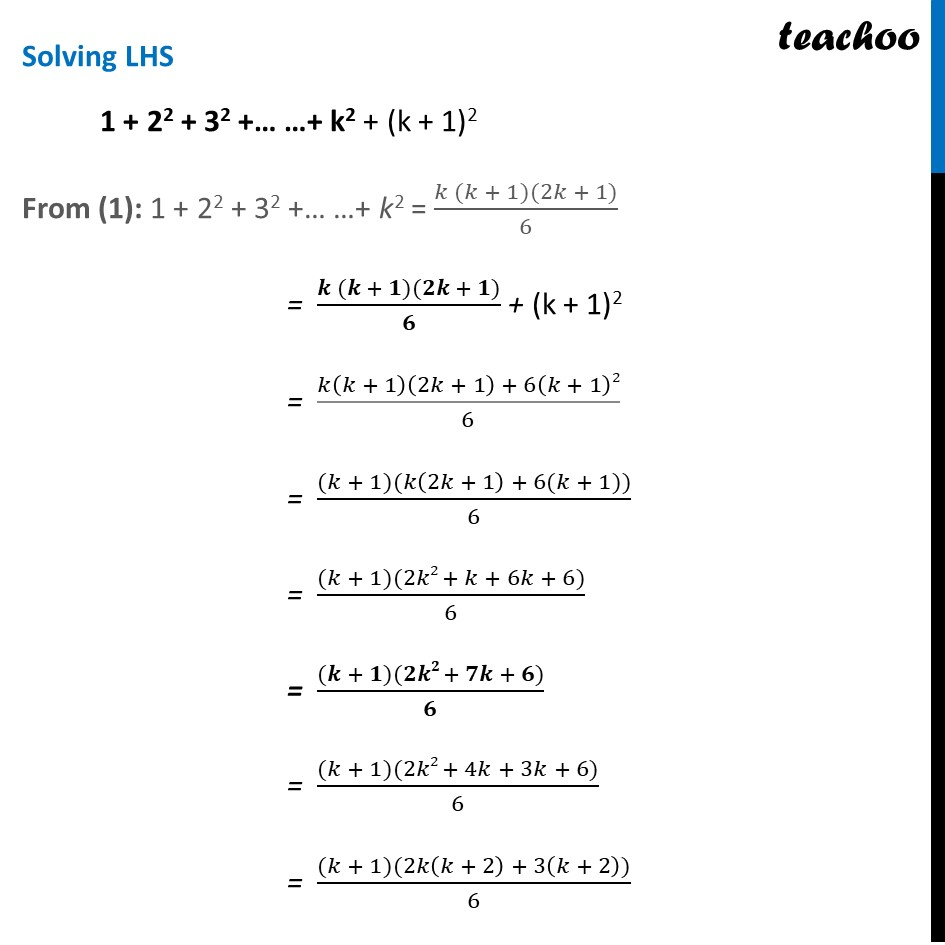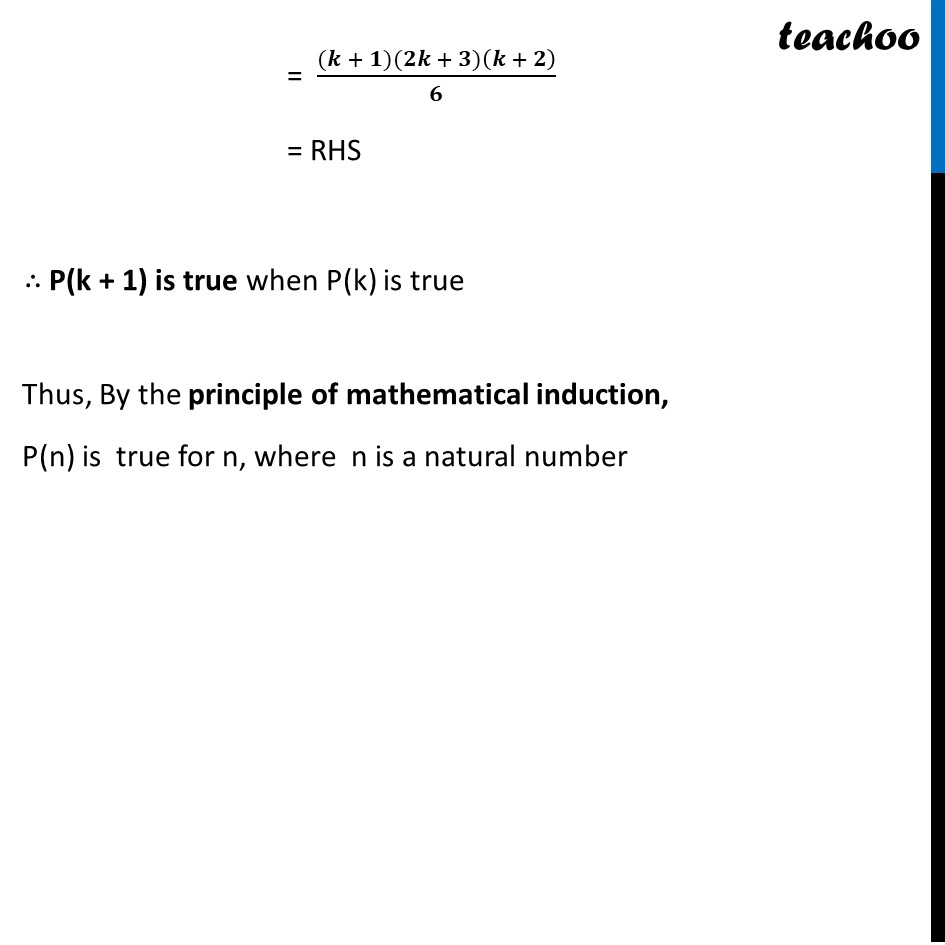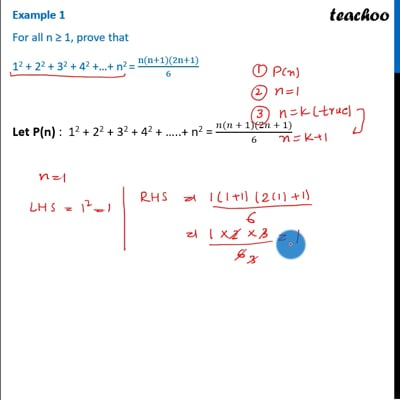This video is only available for Teachoo black users

Solve all your doubts with Teachoo Black (new monthly pack available now!)

### Transcript

Example 1 For all n ≥ 1, prove that 12 + 22 + 32 + 42 +…+ n2 = (n(n+1)(2n+1))/6 Let P(n) : 12 + 22 + 32 + 42 + …..+ n2 = (𝑛(𝑛 + 1)(2𝑛 + 1))/6 Proving for n = 1 For n = 1, L.H.S = 12 = 1 R.H.S = (1(1+1)(2 × 1+ 1))/6 = (1 × 2 × 3)/6 = 1 Since, L.H.S. = R.H.S ∴ P(n) is true for n = 1 Proving P(k + 1) is true if P(k) is true Assume that P(k) is true, P(k): 1 + 22 + 32 +… …+ k2 = (𝑘 (𝑘 + 1)(2𝑘 + 1))/6 We will prove that P(k + 1) is true. P(k + 1): 1 + 22 + 32 +… …+ (k + 1)2 = ((𝑘 + 1)((𝑘 + 1)+ 1)(2 × (𝑘 + 1) +1))/6 P(k + 1): 1 + 22 + 32 +… …+ (k + 1)2 = ((𝑘 + 1)(𝑘 + 2)(2𝑘 + 2 +1))/6 P(k + 1): 1 + 22 + 32 +… …+ k2 + (k + 1)2 = ((𝒌 + 𝟏)(𝒌 + 𝟐)(𝟐𝒌 + 𝟑))/𝟔 We have to prove P(k + 1) is true Solving LHS 1 + 22 + 32 +… …+ k2 + (k + 1)2 From (1): 1 + 22 + 32 +… …+ k2 = (𝑘 (𝑘 + 1)(2𝑘 + 1))/6 = (𝒌 (𝒌 + 𝟏)(𝟐𝒌 + 𝟏))/𝟔 + (k + 1)2 = (𝑘(𝑘 + 1)(2𝑘 + 1) + 6(𝑘 + 1)2)/6 = ((𝑘 + 1)(𝑘(2𝑘 + 1) + 6(𝑘 + 1)))/6 = ((𝑘 + 1)(2𝑘2 + 𝑘 + 6𝑘 + 6))/6 = ((𝒌 + 𝟏)(𝟐𝒌𝟐 + 𝟕𝒌 + 𝟔))/𝟔 = ((𝑘 + 1)(2𝑘2 + 4𝑘 + 3𝑘 + 6))/6 = ((𝑘 + 1)(2𝑘(𝑘 + 2) + 3(𝑘 + 2)))/6 = ((𝒌 + 𝟏)(𝟐𝒌 + 𝟑)(𝒌 + 𝟐))/𝟔 = RHS ∴ P(k + 1) is true when P(k) is true Thus, By the principle of mathematical induction, P(n) is true for n, where n is a natural number